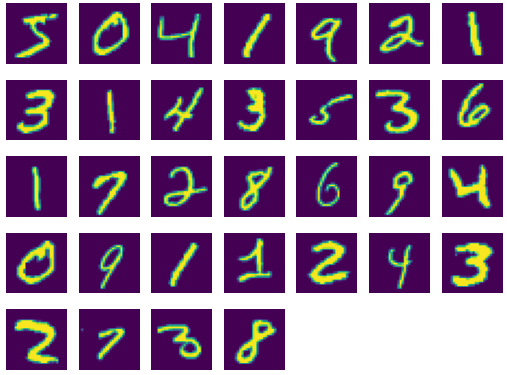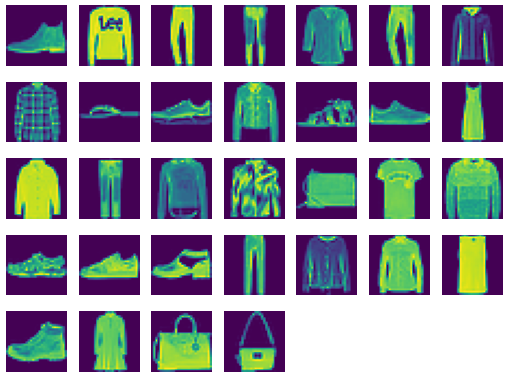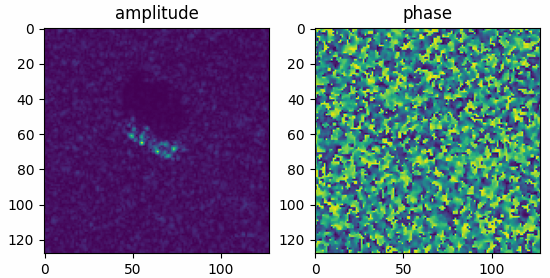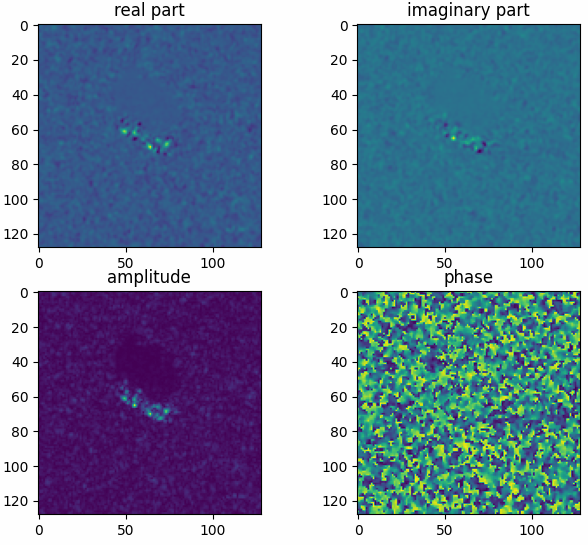# pyaibox.datasets package

## pyaibox.datasets.mnist module

Parameters
• rootdir (str) – root directory path string of mnist dataset

• dataset (str, optional) – dataset to be read, 'test' or 'train', by default 'test'.

• fmt (str, optional) – the dataset formation, 'ubyte' (original) or 'image', by default 'ubyte'.

Returns

Xarray

image data

Yarray

label data

Return type

Examples

Read and show digital MNIST imagesThe results shown in the above figure can be obtained by the following codes.

import pyaibox as pb

rootdir = '/mnt/d/DataSets/oi/dgi/mnist/pics/'
dataset = 'test'
X, Y = read_mnist(rootdir=rootdir, dataset=dataset, fmt='image')
print(X.shape, Y.shape)

rootdir = '/mnt/d/DataSets/oi/dgi/mnist/official/'
dataset = 'train'
X, Y = pb.read_mnist(rootdir=rootdir, dataset=dataset, fmt='ubyte')
print(X.shape, Y.shape)
plt = pb.imshow([X[i] for i in range(0, 32)])
plt.show()

dataset = 'test'
X, Y = pb.read_mnist(rootdir=rootdir, dataset=dataset, fmt='ubyte')
print(X.shape, Y.shape)
plt = pb.imshow([X[i] for i in range(0, 32)])
plt.show()

# output
(10000, 28, 28) (10000,)
(60000, 28, 28) (60000,)
(10000, 28, 28) (10000,)


Read and show Fasion MNIST imagesThe results shown in the above figure can be obtained by the following codes.

import pyaibox as pb

rootdir = '/mnt/d/DataSets/oi/dgi/fashionmnist/official/'
dataset = 'train'
X, Y = pb.read_mnist(rootdir=rootdir, dataset=dataset, fmt='ubyte')
print(X.shape, Y.shape)

plt = pb.imshow([X[i] for i in range(0, 32)])
plt.show()

dataset = 'test'
X, Y = pb.read_mnist(rootdir=rootdir, dataset=dataset, fmt='ubyte')
print(X.shape, Y.shape)

plt = pb.imshow([X[i] for i in range(0, 32)])
plt.show()


## pyaibox.datasets.mstar module

Parameters

filepath (str) – the mstar file path string.

Returns

Return type

dict

Examples

import pyaibox as pb

datapath = pb.data_path('mstar')
filepath = datapath + 'BTR70_HB03787.004'

print(k, v)

pyaibox.datasets.mstar.mstar_raw(filepath, ofmt='c')

Each file is constructed with a prepended, variable-length, Phoenix formatted (ASCII) header which contains detailed ground truth and sensor information for the specific chip. Following the Phoenix header is the data block. The data block is written in Sun floating point format and is divided into two blocks, a magnitude block followed by a phase block. Byte swapping may be required for certain host platforms. Tools for reading and manipulating the header information may be found at https://www.sdms.afrl.af.mil .

Parameters
• filepath (str) – the data file path string.

• ofmt (str, optional) – output data type formation, 'ap' for amplitude and angle, 'c' for complex, and 'r' for real and imaginary.

Returns

the raw data with size $${\mathbb C}^{H\times W}$$ ('c'), $${\mathbb R}^{H\times W \times 2}$$ ('r' or 'ap')

Return type

array

Examples

Read mstar raw amplitude-phase data and show in a figure.The results shown in the above figure can be obtained by the following codes.

import pyaibox as pb
import matplotlib.pyplot as plt

filepath = datapath + 'BTR70_HB03787.004'
x = pb.mstar_raw(filepath, ofmt='ap')
print(x.shape, np.max(x), np.min(x))

plt.figure()
plt.subplot(121)
plt.imshow(x[..., 0])
plt.title('amplitude')
plt.subplot(122)
plt.imshow(x[..., 1])
plt.title('phase')
plt.show()


Read mstar raw complex-valued data and show in a figure.The results shown in the above figure can be obtained by the following codes.

import pyaibox as pb
import matplotlib.pyplot as plt

filepath = datapath + 'BTR70_HB03787.004'
x = pb.mstar_raw(filepath, ofmt='c')
print(x.shape, np.max(x), np.min(x))

plt.figure()
plt.subplot(221)
plt.imshow(x.real)
plt.title('real part')
plt.subplot(222)
plt.imshow(x.imag)
plt.title('imaginary part')
plt.subplot(223)
plt.imshow(np.abs(x))
plt.title('amplitude')
plt.subplot(224)
plt.imshow(np.angle(x))
plt.title('phase')
plt.show()